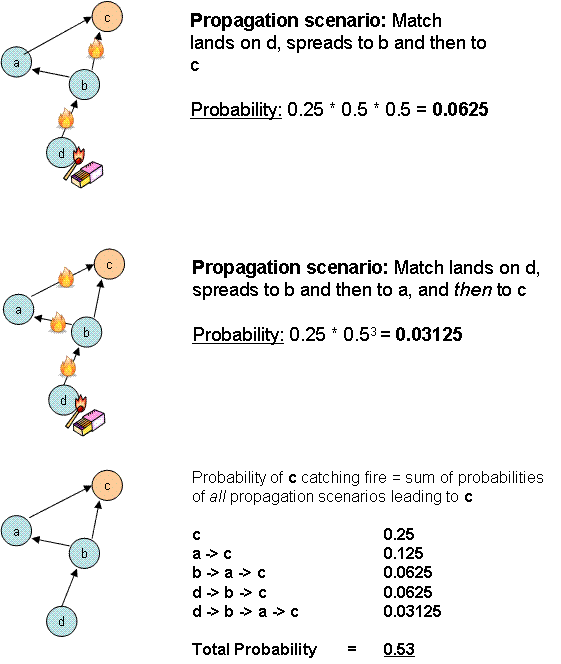## September 12, 2007

...Learn TDD with Codemanship

### Change Propagation Probability Metric

Having slept on it, I think I might now have a bit more insight into the change propagation problem I blogged about yesterday.

If alcohol and other recreational substances haven't yet purged the details from your brain, you may recall that I posited some wierdness about a forest where the trees are in bubbles, and the bubbles can be connected by pipes along which air can flow in one direction only. Burning matches are dropped through holes in the top of the bubbles at random, setting fire to the tree contained within, and there is a finite probability that the fire will spread through the pipes to connected trees, which in turn can spread the fire to trees beyond.

This bizarre thought experiment is designed to model the problem of types in software, dependencies between them, and change propagation along those dependencies.

The first question I asked myself was how could we quantify the flammability of a particular forest? By that, I mean, can we construct a probability distribution that would show us how likely fire is to spread throughout the network of trees?

And over my bran flakes this morning I had a light bulb moment. For each tree in the forest, could we calculate the probability of a match dropped anywhere in the forest causing it to catch fire? To do this, we would have to calculate the sum of probabilities that the fire could travel from every connected tree where the match could land - including the target tree itself, should the match happen to land on it - via every available path between the two trees.

Confused? You will be! Okay, let's do a teeny weeny example to illustrate:

The trick is to consider all of the trees where a fire could start, all of the trees where a fire could end, and all of the available paths between each of those pairs of trees. Let's call these propagation scenarios.If we know the probability that a match will land on a particular tree, and we know the probability that the fire will spread to a connected tree, then we can calculate the probability of a specific propagation scenario. In this very simple example, a match can land on any of 4 trees in the micro-forest, so there's an equal 25% probability that it will land on any specific tree.

Arbitrarily, I have decided that there's a 50% probability of a fire spreading to a connected tree if the air is flowing in that direction. (And a 0% chance if it is flowing in the other direction, of course - fire can only spread in the direction of the airflow, remember.)

To estimate the flammability of the entire forest - all 4 trees - we repeat the exercise for every tree. Then we calculate the average probability of a tree in that forest catching fire.The next question is one of scale. In a city with 7 million people, most Londoners have maybe a dozen real friends, the same as if they lived in a small village. In software, even very large software, most classes have only a handful of nearest neighbours - even if they have a very wide extended neighbourhood. But propagation across 2+ degrees of separation becomes increasingly unlikely, and so maybe increases in the average probability of a change being propagated to any class might be inversely proportional to system size.

I feel a computer simulation coming on...

Of course, it may all turn out to be complete bollocks!

Posted 13 years, 3 months ago on September 12, 2007